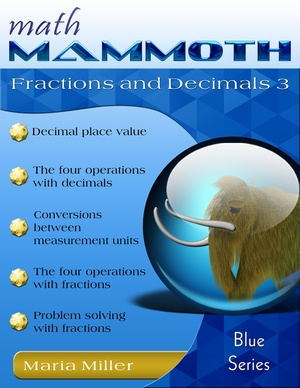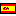^
You are here: HomeBlue Series → Fractions & Decimals 3

# Math Mammoth Fractions & Decimals 3106 pages

## Prices & ordering

Printed copy \$17.65

(credit cards, Google Pay, Paypal, PO)Este libro en español

Math Mammoth Fractions & Decimals 3 continues the study of fraction and decimal topics on the 6th grade level. This book assumes the student already has studied fractions and decimals in the past, for example using Math Mammoth Fractions 2 and Math Mammoth Decimals 2.

The PDF version of this book is enabled for annotation. This means that if you prefer, the student can fill it in on the computer, using the typewriter and drawing tools in Adobe Reader version 9 or greater.

The goal of the book is to go through all of the fraction and decimal arithmetic, using up to six decimal digits and larger denominators in fractions than what is commonly encountered in 4th and 5th grade materials. For some students, this may mean a lot of review, but some students need to restudy these topics if they did not quite master them in earlier grades.

The book starts out with the study of decimals, the metric system, and using decimals in measuring units. If the student already has a good grasp of decimals, consider assigning only 1/3 - 1/2 of the problems, and he should be able to go through those lessons quickly.

We start out by studying place value with decimals and comparing decimals, up to six decimal digits. The next several lessons contain mainly review, just using longer decimals than in 5th grade: adding and subtracting decimals, rounding decimals, using mental math for multiplying and dividing decimals, long division with decimals, fractions to decimals, and multiplying and dividing decimals by the powers of ten.

Scientific notation is a new topic. After that, we turn our attention one more time to dividing decimals by decimals. I have tried to explain the principle behind the common shortcut ("Move the decimal point in both the divisor and the dividend enough steps that the divisor becomes a whole number"). This shortcut actually has to do with the principle that when you multiply the divisor and the dividend by the same number (any number), the value of the quotient does not change. This even ties in with equivalent fractions. Many textbooks never explain this principle in connection with decimal division.

The last lessons in the section with decimals deal with measuring units and the metric system, and nicely round up our study of decimals.

After decimals, the book covers all fraction arithmetic. The goal is that the student will become "fluent" with basic fraction operations, if he isn't already. I assume the student has already studied the four operations of fractions (in 5th grade), so the coverage of this book is slightly quicker and not so in-depth as in Math Mammoth Fractions 1 and Math Mammoth Fractions 2 books.

For example, the lessons don't always delve into the reasons why a certain shortcut works—a lot of that is explained in those two books mentioned. While I consider it very important that the student understands fractions conceptually and understands why certain things are done the way they are done, the material here is building on the material for the earlier grades, where the students have been exposed to those thoughts and ideas.

The lesson Comparing Fraction and Decimal Division is optional. Often, you can solve the same division problem using either fraction or decimal division, if you can convert the numbers from decimals to fractions or vice versa. This lesson just examines the difference between fraction division and decimal division, and best suits advanced students who are interested in it. The page about fraction equations is also optional and can be omitted.

Besides re-studying fraction operations, students also have several problem-solving lessons to study. A lot of the problems in these lessons review and reinforce already studied concepts, such as ratio and using bar diagrams to solve problems with fractional parts. As a new—and hopefully interesting—application, we study scaling in maps.

You might also be interested in:

## Better Yet - Bundle Deals!

*BONUS*: Buy any bundle listed above, and get the fully upgraded Soft-Pak software for FREE (includes 4 math and 2 language arts programs, complete with on screen and printable options).

## How and where to order

You can buy Math Mammoth books at:

• Here at MathMammoth.com website — simply use the "Add to cart" buttons you see on the product pages.
• Rainbow Resource carries printed copies for the Light Blue series books, plus several CDs (Light Blue and Blue series).
• At Teachers Pay Teachers you can purchase the Light Blue Series downloads, plus topical units.
• Lulu sells printed copies for most of the Math Mammoth materials (various series).

By purchasing any of the books, permission IS granted for the teacher (or parent) to reproduce this material to be used with his/her students in a teaching situation; not for commercial resale. However, you are not permitted to share the material with another teacher.

In other words, you are permitted to make copies for the students/children you are teaching, but not for other teachers' usage.

Math Mammoth books are PDF files. You will need Adobe Reader to view them, including if you use a Mac or Linux. You can try other PDF viewers, but they seem to either omit or mess up some of the images.
WAIT!

Receive my monthly collection of math tips & resources directly in your inbox — and get a FREE Math Mammoth book!You can unsubscribe at any time.

### Math Mammoth Tour

Confused about the different options? Take a virtual email tour around Math Mammoth! You'll receive:

An initial email to download your GIFT of over 400 free worksheets and sample pages from my books. Six other "TOURSTOP" emails that explain the important things and commonly asked questions concerning Math Mammoth curriculum. (Find out the differences between all these different-colored series!)

This way, you'll have time to digest the information over one or two weeks, plus an opportunity to ask me personally about the curriculum.
A monthly collection of math teaching tips & Math Mammoth updates (unsubscribe any time)### "Mini" Math Teaching Course

This is a little "virtual" 2-week course, where you will receive emails on important topics on teaching math, including:

- How to help a student who is behind
- Troubles with word problems
- Teaching multiplication tables
- Why fractions are so difficult
- The value of mistakes
- Should you use timed tests
- And more!

A GIFT of over 400 free worksheets and sample pages from my books right in the very beginning.A monthly collection of math teaching tips & Math Mammoth updates (unsubscribe any time)
Enter your email to receive math teaching tips, resources, Math Mammoth news & sales, humor, and more! I tend to send out these tips about once monthly, near the beginning of the month, but occasionally you may hear from me twice per month (and sometimes less often).• A GIFT of over 400 free worksheets and sample pages from my books.